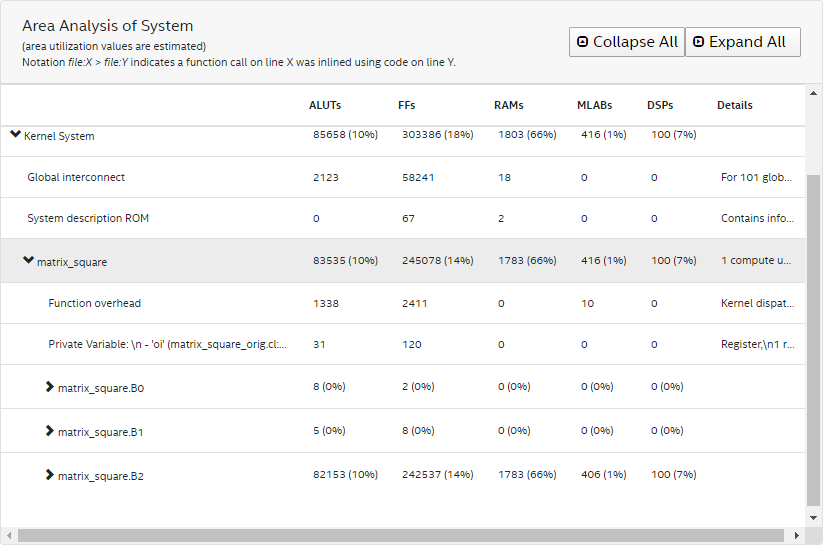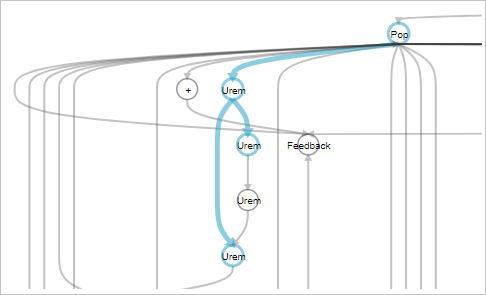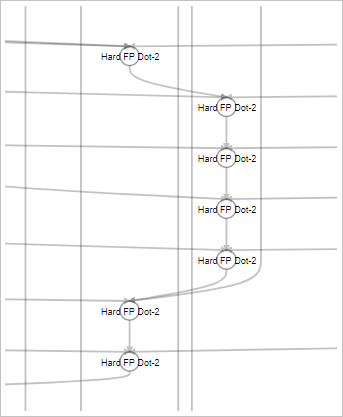ID 683521
Date 12/19/2022
Public

## 2.7. Optimizing an OpenCL Design Example Based on Information in the HTML Report

A guide on how to use the information in the HTML report to optimize an OpenCL™ kernel.

OpenCL design example that performs matrix square AxA:

 // performs matrix square A*A
// A is a square LEN*LEN matrix
// A = [ r0       : [ c, c, ... c[LEN-1] ],
//       r1       : [ c, ...                ],
//       ...                                   ],
//       r[LEN-1] : [                          ] ]

// LEN = 100

kernel void matrix_square (global float* restrict A, global float* restrict out)
{
for( unsigned oi = 0 ; oi < LEN*LEN ; oi++ )
{
float sum = 0.f;
int row = oi / LEN;
int col = oi % LEN;

#pragma unroll
for ( int stride = 0 ; stride < LEN ; stride++ )
{
unsigned i = (row * LEN) + stride;
unsigned j = (stride * LEN) + col;
sum += A[i] * A[j];
}

out[oi] = sum;
}
}

The area analysis of the kernel matrix_square indicates that the estimated usages of flipflops (FF) and RAMs are high.

Figure 25. Area Report of the Unoptimized Kernel matrix_squareFurther examination of block 2 in the System viewer shows that Block2 also has a high latency value.

Figure 26. System Viewer Results for the Unoptimized Kernel matrix_squareThe cause for these performance bottlenecks is the system is loading data from global memory from inside a loop. Therefore, the first optimization step you can take is to preload the data into local memory, as shown in the following modified code:

 // 1. preload the data into local memory

kernel void matrix_square_v1 (global float* restrict A, global float* restrict out)
{
local float cache_a[LEN*LEN];
for( unsigned k = 0 ; k < LEN*LEN ; k++ )
{
cache_a[k] = A[k];
}

for( unsigned oi = 0 ; oi < LEN*LEN ; oi++ )
{
float sum = 0.f;
int row = oi / LEN;
int col = oi % LEN;

#pragma unroll
for( unsigned stride = 0 ; stride < LEN ; stride++ )
{
unsigned i = (row * LEN) + stride;
unsigned j = (stride * LEN) + col;
sum += cache_a[i] * cache_a[j];
}

out[oi] = sum;
}
}
Figure 27. Area Report Results for the Modified Kernel matrix_square_v1Figure 28. Cluster View of the Modified Kernel matrix_square_v1If you remove the modulus computation and replace it with a column counter, as shown in the modified kernel matrix_square_v2, you can reduce the amount of adaptive look-up table (ALUT) and FF use.

 // 1. preload the data into local memory
// 2. remove the modulus computation

kernel void matrix_square_v2 (global float* restrict A, global float* restrict out)
{
local float cache_a[LEN*LEN];
for( unsigned k = 0 ; k < LEN*LEN ; k++ )
{
cache_a[k] = A[k];
}

unsigned row = 0;
unsigned col = 0;

for( unsigned oi = 0 ; oi < LEN*LEN ; oi++ )
{
float sum = 0.f;

// keep a column counter to know when to increment row
if( col == LEN - 1 )
{
col = 0;
row += 1;
}
else
{
col += 1;
}

#pragma unroll
for( unsigned stride = 0 ; stride < LEN ; stride++ )
{
unsigned i = (row * LEN) + stride;
unsigned j = (stride * LEN) + col;
sum += cache_a[i] * cache_a[j];
}

out[oi] = sum;
}
}
Figure 29. Area Report of Kernel matrix_square_v2Figure 30. Cluster View of Kernel matrix_square_v2Further examination of the area report of matrix_square_v2 reveals that the computations for indexes i and j (that is, unsigned i = (row * LEN) + stride and unsigned j = (stride * LEN) + col, respectively) have very different ALUT usage estimations.

A way to optimize DSP and RAM block usages for index calculation is to remove the multiplication computation and simply keep track of the addition, as shown in the modified kernel matrix_square_v3 below.

// 1. preload the data into local memory
// 2. remove the modulus computation
// 3. remove DSP and RAM blocks for index calculation helps reduce the latency

kernel void matrix_square_v3 (global float* restrict A, global float* restrict out)
{

local float cache_a[LEN*LEN];
for( unsigned k = 0 ; k < LEN*LEN ; k++ )
{
cache_a[k] = A[k];
}

unsigned row_i = 0;
unsigned row_j = 0;

for( unsigned oi = 0 ; oi < LEN*LEN ; oi++ )
{
unsigned i, j;

// keep a column base counter to know when to increment the row base
if( row_j == LEN - 1 )
{
row_i += LEN;
row_j = 0;
}
else
{
row_j += 1;
}

// initialize i and j
i = row_i;
j = row_j;

float sum = 0.f;
#pragma unroll
for( unsigned stride = 0 ; stride < LEN ; stride++ )
{
i += 1;       // 0, 1, 2, 3, 0,...
j += LEN;     // 0, 3, 6, 9, 1,...
sum += cache_a[i] * cache_a[j];
}

out[oi] = sum;
}
}

By removing the multiplication step, you can reduce DSP usage as shown in the area report below. In addition, the modification helps reduce latency.

Figure 31. Area Report of Kernels matrix_square_v3Figure 32. System Viewer of the Kernel matrix_square_v3To resolve the loop-carried dependency, unroll the sum-product for complete parallelism and create registers to avoid multiple copies of cache_a, as shown in the code below in the modified kernel matrix_square_v4.

// 1. preload the data into local memory
// 2. remove the modulus computation
// 3. remove DSP and RAM blocks for index calculation helps reduce the latency
// 4. unroll the sum-product for full parallelism, create registers to avoid many copies of cache_a

kernel void matrix_square_v4 (global float* restrict A, global float* restrict out)
{

local float cache_a[LEN*LEN];
for( unsigned k = 0 ; k < LEN*LEN ; k++ )
{
cache_a[k] = A[k];
}

unsigned row_i = 0;
unsigned row_j = 0;

for( unsigned oi = 0 ; oi < LEN*LEN ; oi++ )
{
unsigned i, j;
// keep a column base counter to know when to increment the row base
if( row_j == LEN - 1 )
{
row_i += LEN;
row_j = 0;
}
else
{
row_j += 1;
}

// initialize i and j
i = row_i;
j = row_j;

float r_buf[LEN];
float c_buf[LEN];
for( int stride = 0 ; stride < LEN ; stride++ )
{
i += 1;       // 0, 1, 2, 3, 0,...
j += LEN;     // 0, 3, 6, 9, 1,...
r_buf[stride] = cache_a[i];
c_buf[stride] = cache_a[j];
}

// uses harder floating point when -fp-relaxed is used
float sum = 0.f;
#pragma unroll
for(unsigned idx = 0; idx &lt; LEN; idx++)
{
sum += r_buf[idx] * c_buf[idx];
}

out[oi] = sum;
}
}

As shown in the cluster view results below, by breaking up the computation steps, you can achieve higher throughput at the expense of increased area usage. The modification also reduces the latency by 50%.

Figure 33. Cluster View of the Modified Kernel matrix_square_v4The following cluster view provides an alternative with -fp-relaxed to show dot product instead of chain:

Figure 34. Cluster View of the Modified Kernel matrix_square_v4The following table provides the throughput comparison for all five versions of the kernel:

Table 8.  Throughput Comparison
Kernel ALUTs FFs RAMs MLABs DSPs Dot Product Loop Latency
matrix_square 81806 (10%) 302792 (18%) 1989 (73%) 408 (1%) 100 (7%) 637
matrix_square_v1 20094 (2%) 38814 (2%) 1619 (60%) 248 (1%) 100 (7%) 380
matrix_square_v2 15487 (2%) 51813 (3%) 1110 (41%) 298 (1%) 100 (7%) 364
matrix_square_v3 18279 (2%) 37554 (2%) 1618 (60%) 244 (1%) 100 (7%) 362
matrix_square_v4

(-fp-relaxed)

9681 (1%) 22409 (1%) 257 (9%) 67 (0%) 103 (7%) 37